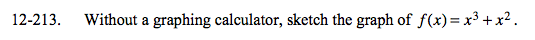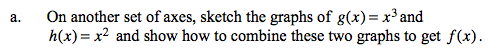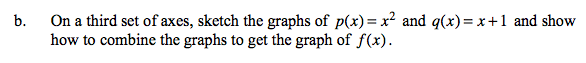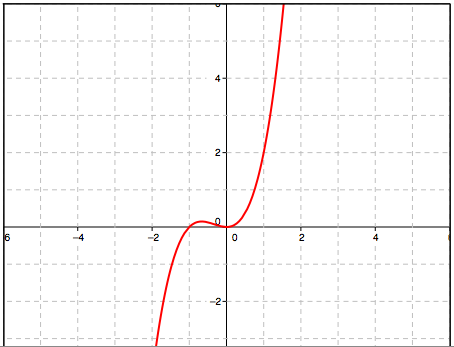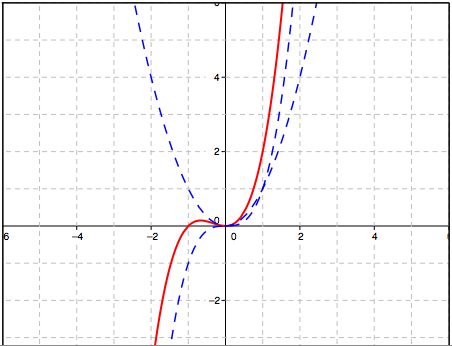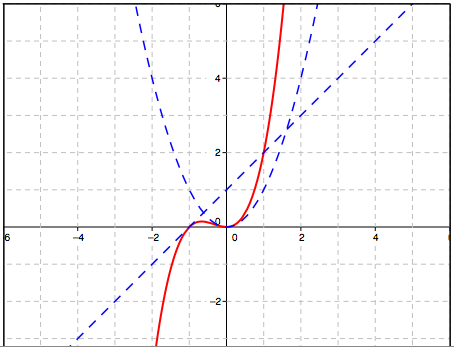### Home > A2C > Chapter 12 > Lesson 12.5.2 > Problem12-213

12-213.
1. Without a graphing calculator, sketch the graph of f (x) = x3 + x2. Homework Help ✎1. On another set of axes, sketch the graphs of g(x) = x3 and h(x) = x2 and show how to combine these two graphs to get f(x).

2. On a third set of axes, sketch the graphs of p(x) = x2 and q(x) = x + 1 and show how to combine the graphs to get the graph of f(x).